# Watts / Volts / Amps / Ohms calculator

Watts (W) - volts (V) - amps (A) - ohms (Ω) calculator.

Calculates power / voltage / current / resistance.

Enter 2 values to get the other values and press the Calculate button:

 Enter watts: micro-watts (μW) milli-watts (mW) watts (W) kilo-watts (kW) mega-watts (MW) Enter volts: micro-volts (μV) milli-volts (mV) volts (V) kilo-volts (kV) mega-volts (MV) Enter amps: micro-amps (μA) milli-amps (mA) amps (A) kilo-amps (kA) mega-amps (MA) Enter ohms: ohms (Ω) kilo-ohms (kΩ) mega-ohms (MΩ)

### Ohms calculations

The resistance R in ohms (Ω) is equal to the voltage V in volts (V) divided by the current I in amps (A):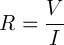The resistance R in ohms (Ω) is equal to the squared voltage V in volts (V) divided by the power P in watts (W):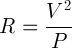The resistance R in ohms (Ω) is equal to the power P in watts (W) divided by the squared current I in amps (A):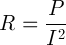### Amps calculations

The current I in amps (A) is equal to the voltage V in volts (V) divided by the resistance R in ohms (Ω):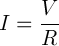The current I in amps (A) is equal to the power P in watts (W) divided by the voltage V in volts (V):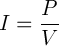The current I in amps (A) is equal to the square root of the power P in watts (W) divided by the resistance R in ohms (Ω):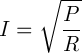### Volts calculations

The voltage V in volts (V) is equal to the current I in amps (A) times the resistance R in ohms (Ω):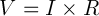The voltage V in volts (V) is equal to the power P in watts (W) divided by the current I in amps (A):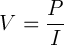The voltage V in volts (V) is equal to the square root of the power P in watts (W) times the resistance R in ohms (Ω):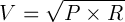### Watts calculation

The power P in watts (W) is equal to the voltage V in volts (V) times the current I in amps (A):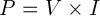The power P in watts (W) is equal to the squared voltage V in volts (V) divided by the resistance R in ohms (Ω):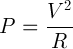The power P in watts (W) is equal to the squared current I in amps (A) times the resistance R in ohms (Ω):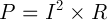Ohm's law ►